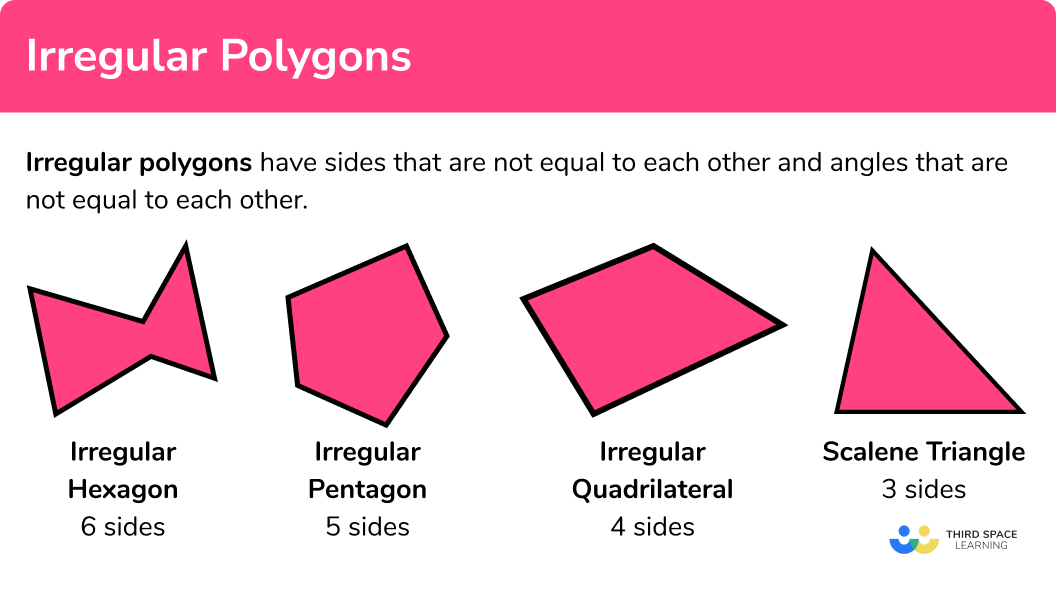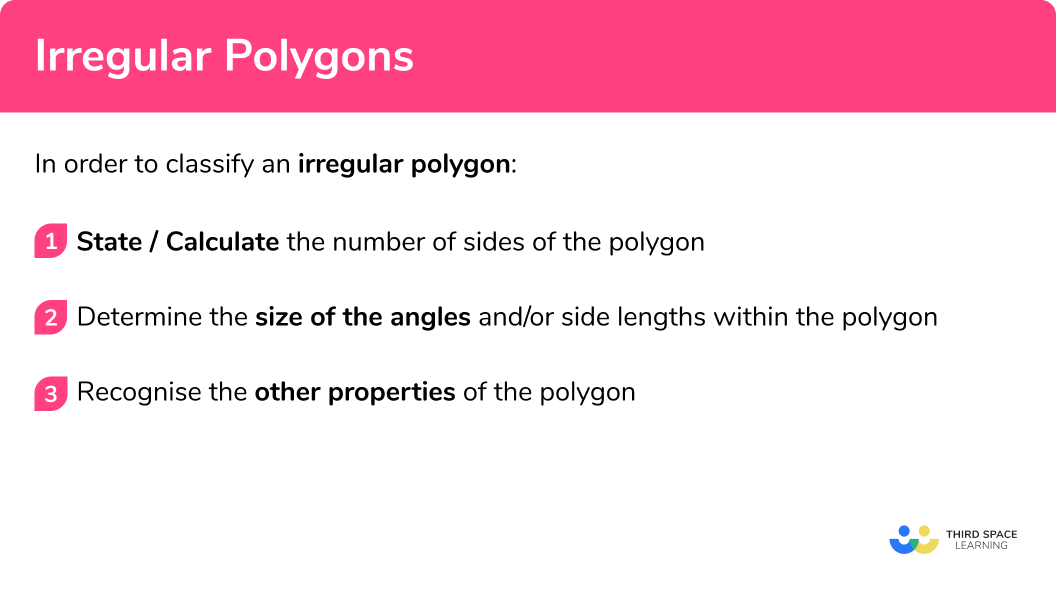# Irregular Polygons

Here we will learn about polygons, including regular polygons, angles in polygons, and complex polygons.

There are also polygons worksheets based on Edexcel, AQA and OCR exam questions, along with further guidance on where to go next if you’re still stuck.

## What is an irregular polygon?

An irregular polygon is a 2D shape that has straight sides that are not equal to each other and angles that are not equal to each other. You need to be able to classify geometric shapes based on their properties and sizes and find unknown angles in any polygon. To do this, we need to look closely at the properties of these shapes.

E.g.

To do this, we need to look closely at the properties of these shapes.

### What is an irregular polygon?### Properties of irregular polygons

• Irregular polygons have sides that are not equal to each other or angles that are not equal to each other or both.
• The table below gives the name of several irregular polygon.
• The sum of interior angles can be calculated using the formula:

Sum of interior angles = (n-2) × 180^{\circ}

where n represents the number of sides.

As the size of each angle is not equal, we can determine the size of each angle by adding together the interior angles that we know and putting it equal to the sum of the interior angles, then solving the equation.

• Exterior angles are supplementary to the interior angle.

Sum of exterior angles of a polygon = 360°

We can use this property to find either the interior angle, or exterior angle at a vertex.

As the size of each angle is not equal, we can determine the size of an exterior angle by adding together the exterior angles that we know and subtracting it from 360° .

• Irregular polygons can be either convex polygons or concave polygons

A convex polygon is a polygon with all the interior angles less than 180° . A concave polygon has at least one angle that is greater than 180° (or a reflex angle).

E.g.

## How to classify an irregular polygon

In order to classify an irregular polygon:

1. State/calculate the number of sides of the polygon.
2. Determine the size of the angles and side lengths within the polygon.
3. Recognise the other properties of the polygon.

### How to classify an irregular polygon### Related lessons on polygons

Irregular polygon is part of our series of lessons to support revision on polygons. You may find it helpful to start with the main polygons lesson for a summary of what to expect, or use the step by step guides below for further detail on individual topics. Other lessons in this series include:

## Irregular polygons examples

### Example 1: irregular triangles

Given that ABC is an isosceles triangle and BCD is a straight line, classify the polygon ABD .

1. State/calculate the number of sides of the polygon.

ABC has 3 sides and so it is a triangle.

2Determine the size of the angles and/or side lengths within the polygon.

As BCA = 116° , angle ACD = 180 - 116 = 64° .

As ABC is an isosceles triangle, ABC = BAC = (180 - 116)\div 2 = 32° .

As angles in a triangle total 180° , angle CAD = 180 - (74 + 64) =  42° .

Angle BAD = 42 + 32 = 74° .

3Recognise the other properties of the polygon.

As the three angles in the triangle ABC are 74°, 74° and 32° . The triangle ABD has two equal angles and so it is isosceles.

The polygon is an isosceles triangle, which is an irregular polygon.

Classify the following polygon:

State/calculate the number of sides of the polygon.

Determine the size of the angles and/or side lengths within the polygon.

Recognise the other properties of the polygon.

### Example 3: types of trapezium

The polygon below is a quadrilateral. By using angle properties, determine the type of quadrilateral.

State/calculate the number of sides of the polygon.

Determine the size of the angles and/or side lengths within the polygon.

Recognise the other properties of the polygon.

### Example 4: pentagon

A pentagon is constructed by joining an isosceles triangle with a rectangle. The line AM is a line of symmetry. Show that the polygon is irregular.

State/calculate the number of sides of the polygon.

Determine the size of the angles and/or side lengths within the polygon.

Recognise the other properties of the polygon.

### Example 5: concave polygon

Below is a description of a polygon. From the description, determine the classification of the polygon.

The polygon has 10 edges.

The polygon has a rotational symmetry of order 5 .

The interior angles are equal to 42° or 246° .

The polygon can be constructed from 5 congruent kites.

State/calculate the number of sides of the polygon.

Determine the size of the angles and/or side lengths within the polygon.

Recognise the other properties of the polygon.

### Example 6: algebraic

The interior angles of a polygon are: 4x+16, 6x+8 and 2x+12 . Classify the polygon.

State/calculate the number of sides of the polygon.

Determine the size of the angles and/or side lengths within the polygon.

Recognise the other properties of the polygon.

### Common misconceptions

• Angles in polygons

Make sure you know your angle properties. Getting these confused causes quite a few misconceptions.

• Angles in a triangle total 180°
• Angles in a quadrilateral total 360°
• Angles on a straight line total 180°

There are many quadrilaterals and it is common to confuse the properties, especially for a rhombus, parallelogram, or trapezium.

As well as this, stating that the polygon is a quadrilateral is not enough information for a classification.

• Incorrect assumptions for triangles

Assuming a triangle is isosceles or equilateral can have an impact on the size of angles within the rest of the polygon, so make sure you can explain why you have chosen a specific type of triangle.

As well as this, stating that the polygon is a triangle is not enough information for a classification. You must state whether it is isosceles/ equilateral etc.

### Practice Irregular polygons questions

1. BD is parallel to AE in the triangle ACE . Classify the polygon BCDEquilateral triangleRight Angle triangleIsosceles triangleScalene triangleThe angle BDC = 180 – 127 = 53^{\circ} (angles on a straight line total 180^{\circ} ).
Angle CBD = 180 – (65 + 53) = 62^{\circ} (angles in a triangle total 180^{\circ} ).
All angles of BCD are different.

2. A quadrilateral is inscribed in a circle with centre O . The diagonals meet perpendicularly at the point E where AC = 2AE . Use circle theorems to determine the classification of the quadrilateral ABCD .DiamondIsosceles triangleKiteAs AC = 2AE , and BD is perpendicular to AC , triangles ABC and ADC are isosceles triangles. This means that the two angles at A and C are equal and the side lengths AB and BC , and AD and CD are equal. This is a kite.

3. The polygon below has an order of rotational symmetry of 4 . Classify the polygon.OctagonRegular octagonRegular pentagonAs the order of rotational symmetry is 4 , the angles at A, C, E and G are all equal to 40^{\circ} , and the angles at B, D, F, and H are equal to 360 – 130 = 230^{\circ} . The polygon has 8 sides but the interior angles are not equal.

4. 6 congruent triangles are joined together to form a 6 sided polygon. Each line segment within the polygon is the same length. The new polygon has two acute angles, 3 obtuse angles and one reflex angle. The polygon has one line of symmetry and no rotational symmetry. Classify the polygon.Regular hexagonHexagonParallelogramAs the polygon has 6 sides and different interior angles, the polygon is a hexagon.

5. The polygon below is incomplete. Given that the polygon is made up of 10 congruent kites, classify the polygon.Regular decagonRegular dodecagonRegular icosagonRegular octagonAs the polygon is made of 10 congruent kites, each side length is the same and the interior angles are also equal due to the kite also being symmetrical.

6. The interior angles of a polygon are: 12x-30, 8x+40, 7x+30, 8x, and 5x+20 . The sum of the angles is equal to 540^{\circ} . Classify the polygon.

PentagonHexagonRegular pentagonRegular hexagon12x-30+8x+40+7x+30+8x+5x+20=540\\ 40x+60=540\\ 40x=480\\ x=12

As x=12\\ 12x-30=12\times12-30=114^{\circ}\\ 8x+40=8\times12+40=136^{\circ}\\ 7x+30=7\times12+30=114^{\circ}\\ 8x=8\times12=96^{\circ}\\ 5x+20=5\times12+20=80^{\circ}

The internal angles are not equal and so the polygon is a pentagon.

### Irregular polygons GCSE questions

1. (a) An isosceles trapezium is made when you join two triangles together along an edge with the same length. ABC is an isosceles triangle. Work out the size of the angle ACD .(b) What type of triangle is ACD ?

(5 marks)

(a)
BCA = (180 – 116)\div2 = 32^{\circ}

(1)

CAD = 116 – 32 = 84^{\circ}

(1)

ADC = 180 – 116 = 64^{\circ}

(1)

ACD = 180 – (84+64) = 32^{\circ}

(1)

(b)
Scalene

(1)

2. (a) Show that the triangle is a right angle triangle.(b)The area of both polygons are equal. Calculate the length x .

(5 marks)

(a)
5^{2}+12^{2}=25+144=169

(1)

\sqrt{169}=13

(1)

The sum of the square of the two shorter sides is equal to the square of the hypotenuse so the triangle contains a right angle

(1)

(b)
Triangle area = 5\times12\div2=30cm^{2}

(1)

30\div6=x\\ x=5cm

(1)

3. (a) The polygon ABC is half of a kite where AC is the longest length of the kite. Write an expression for the area of the kite.(b) Given that the area of the smaller triangle ABO = 40cm^{2} , calculate the area of the kite.
(c) Calculate the size of angle OAB .

(5 marks)

(a)
8x^{2}

(1)

(b)
x^{2}=40

(1)

8x^{2}=320cm^{2}

(1)

(c)
OAB=tan^{-1}(\frac{2x}{x})

(1)

OAB = 63.4^{\circ}

(1)

## Learning checklist

You have now learned how to:

• Plot specified points and draw sides to complete a given polygon
• Distinguish between regular and irregular polygons based on reasoning about equal sides and angles
• Compare and classify geometric shapes based on their properties and sizes and find unknown angles in any triangles, quadrilaterals, and regular polygons
• Describe, sketch and draw using conventional terms and notations: points, lines, parallel lines, perpendicular lines, right angles, regular polygons, and other polygons that are reflectively and rotationally symmetric
• Derive and use the sum of angles in a triangle and use it to deduce the angle sum in any polygon, and to derive properties of regular polygons

## Still stuck?

Prepare your KS4 students for maths GCSEs success with Third Space Learning. Weekly online one to one GCSE maths revision lessons delivered by expert maths tutors.

Find out more about our GCSE maths tuition programme.